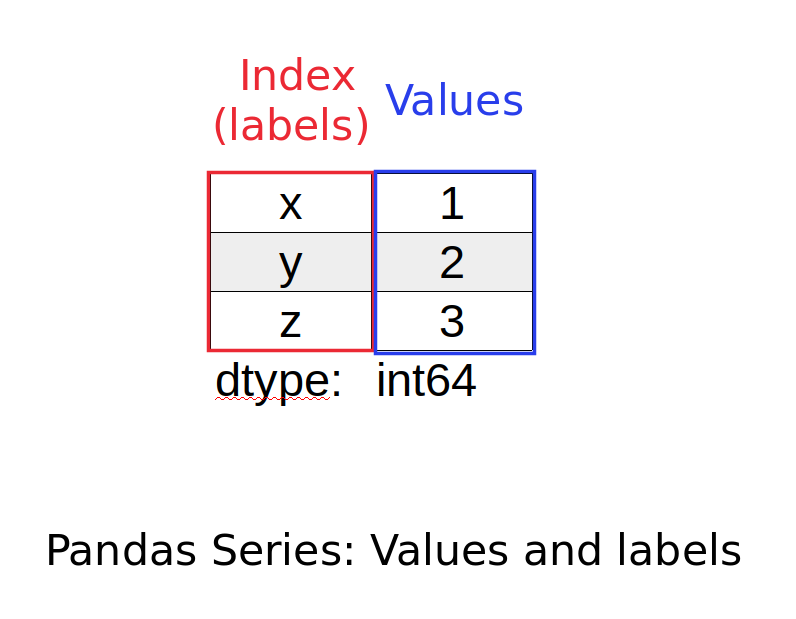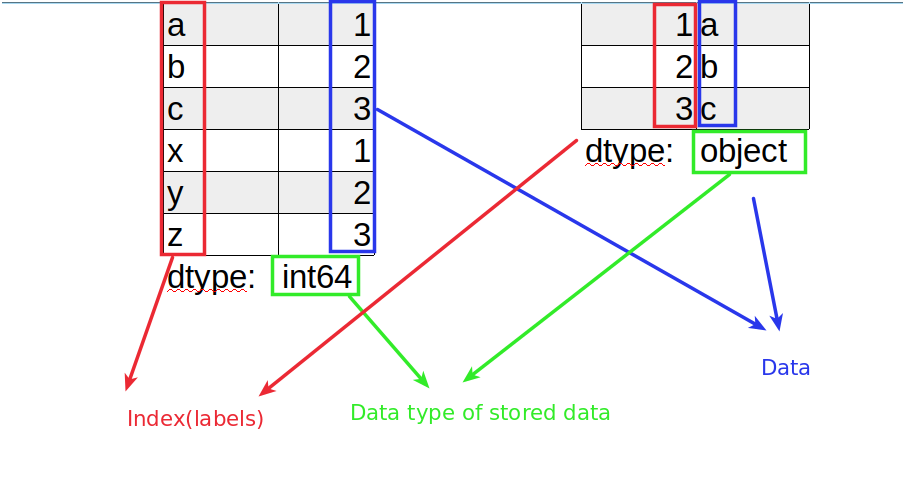In this guide, we'll learn about one of the two main data structures in Pandas - Series. Our goal will be to understand the usage of this structure.

Understanding what a Pandas Series will avoid simple mistakes in future. Pandas Series play a major role in data wrangling and transformation.

## Step 1: What is Pandas Series

There two main data structures in Pandas:

The official documentation describes Series like:

One-dimensional ndarray with axis labels (including time series).

So Series is a one-dimensional array. It has labels to access data.

You can imagine it like a sequence of post boxes - each has an address and can store different items.

Note:

What is ndarray? `ndarray` is a multi-dimensional array in Numpy. It should have homogeneous and fixed-size items.

The image below illustrates the Series visually. There are two main parts:

• labels (also index or axis=0) - it can be set explicitly ot auto generated
• data (also - values) - can store different types of data and even empty values like null, None, NANote:

Labels must be a hashable type (no need to be unique).

Series is the building block for DataFrames.

## Step 2: Create a Pandas Series

In order to create Pandas Series we can use the following constructor:

``````class pandas.Series(data=None, index=None, dtype=None, name=None, copy=False, fastpath=False)
``````

Below you can find example of creating Series from a dict:

``````import pandas as pd

d = {'x': 1, 'y': 2, 'z': 3}
s = pd.Series(data=d, index=['x', 'y', 'z'])
``````

In the example above we have labeled data:

`d = {'x': 1, 'y': 2, 'z': 3}`

and index(which should match):

`index=['x', 'y', 'z']`

This would result into:

``````x    1
y    2
z    3
dtype: int64
``````

If the index is skipped:

``````d = {'x': 1, 'y': 2, 'z': 3}
s = pd.Series(data=d)
``````

result would be the same:

``````x    1
y    2
z    3
dtype: int64
``````

and if we change order of the index:

``````d = {'x': 1, 'y': 2, 'z': 3}
s = pd.Series(data=d, index=['x', 'z', 'y'])
``````

we will get different order in the Series:

``````x    1
z    3
y    2
dtype: int64
``````
Note:

If data is dict-like and index is None, then the keys in the data are used as the index.

If the index is not None, the resulting Series is reindexed with the index values.

As you can see the Series has a dtype. Dtype represents the type of the stored data.Since in the above we store integers Pandas creates the Series with `dtype: int64`.

## Step 3: Create Pandas Series from list

We can create Pandas Series also by providing iterable like a list:

``````s = pd.Series(['a', 'b', 'c'])
``````

This time the dtype is object:

``````0    a
1    b
2    c
dtype: object
``````

If index is not provided as in the example with the dict - then automatic labels will be applied on the Series starting from 0.

## Step 4: Pandas Series Attributes/Properties

Attributes pr properties of Series are storing important information or performing features. Attributes can be invoked in this way:

``````s.dtype
``````

which will result into:

`dtype('int64')`

Below you can find some of the most used Series attributes.

Let have the next Series:

``````x    1
y    2
z    3
dtype: int64
``````

Below you can find the attribute, the explanation and the result of the execution.

• dtype

Return the dtype object of the underlying data.

Example result: `dtype('int64')`

• index

The index (axis labels) of the Series.

Example result: `Index(['x', 'y', 'z'], dtype='object')`

• values

Return Series as ndarray or ndarray-like depending on the dtype.

Example result: `array([1, 2, 3])`

• shape

Return a tuple of the shape of the underlying data.

Example result: `(3,)`

• loc

Access a group of rows and columns by label(s) or a boolean array.

``````s.loc[]
``````

Example result:

``````1    b
dtype: object
``````

## Step 5: Series Methods in Pandas

The core functionality of Pandas is available via methods. In this section you can find some of the most popular Series methods.

Currently the official documentation shows around 200 Pandas Series methods! Usually the difference between attributes and methods is the brackets - `()`

Let use the next Series and check the methods:

``````x    1
y    2
z    3
dtype: int64
``````

Example usage of Series methods:

``````s.sum()
``````
• sum

Return the sum of the values over the requested axis.

Example result: `6`

• head([n]) Similar one is `tail()` which returns the last n rows.

Return the first n rows.

`s.head(2)`:

result:

``````x    1
y    2
dtype: int64
``````
• isna()

Detect missing values.

Example result:

``````x    False
z    False
y    False
dtype: bool
``````
• duplicated([keep])

Indicate duplicate Series values.

Example result:

``````x    False
z    False
y    False
dtype: bool
``````

fillna([value, method, axis, inplace, ...])

Fill NA/NaN values using the specified method.

• sort_values([axis, ascending, inplace, ...])

Sort by the values.

For example:

``````s.sort_values(ascending=False)
``````

will return sorted Series:

z 3
y 2
x 1
dtype: int64

Note:

The original Series will not be changed. The copy of it will be returned.

## Step 6: Alias Methods of Pandas Series

Those are special aliases for methods like String or Datetime. The idea is to use methods from spaces like: `StringMethods`.

Example usage is:

``````s.str.count('a')
``````

### Pandas Series .str

alias of pandas.core.strings.accessor.StringMethods

Some of the StringMethods are:

Example of using `.str` accessor in real life: How to Replace Regex Groups in Pandas

### Pandas Series .dt

alias of pandas.core.indexes.accessors.CombinedDatetimelikeProperties

Some properties are:

Example of using `.dt` accessor in real life: How to Extract Month and Year from DateTime column in Pandas

Note:

You can use those aliases only if the data has a specific type. Otherwise you will get an error:

For example accessing `.str` on integer values will raise `AttributeError`:

AttributeError: Can only use .str accessor with string values!